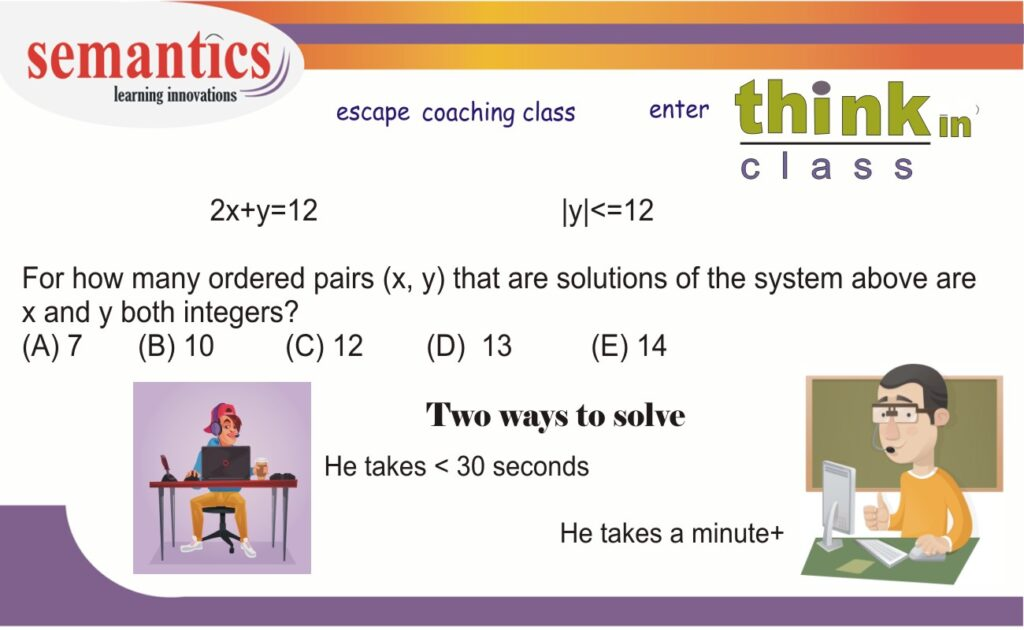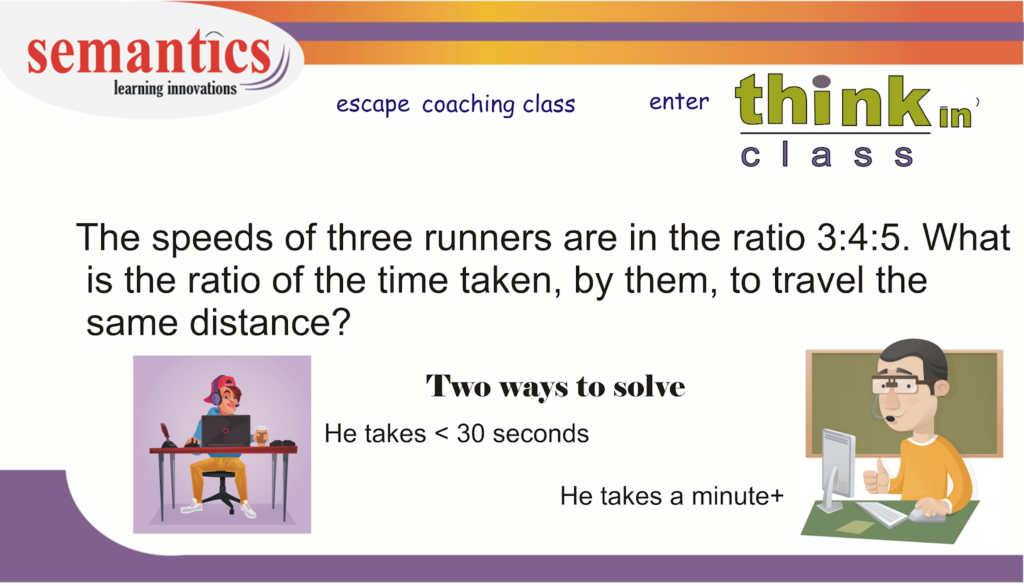# math problem solving skills – 11

The quantitative reasoning section tests your logical skills as well as your knowledge of math concepts.  To score high, you need to remember various formulas, theorems. Also you need to master critical problem-solving skills.

Today I am going to  take you through one problem -solving skill – counting

Lets take this math problem

Observe the problem solving process…## Method 1: Conventional approach2x + y = 12

x= (12 – y)/2

x= 6 – (y/2)

Y is an integer. So you need to list numbers which satisfy |y|<=12. The range of y values are from -12 to 12.

So lets list the pairs

The ordered pairs of (x, y)would be:

• (12, -12)
• (11, -10)
• (10, -8)
• (9, -6)
• (8, -4)
• (7, -2)
• (6, 0)
• (5, 2)
• (4, 4)
• (3, 6)
• (2, 8)
• (1, 10)
• (0, 12)

13 pairs.

## Method 2: Visualization2x + y = 12
y=12-2x

|y|<=12

Hence
|12-2x|<=12
-12 <=12-2x <=12

Dividing by 2
-6 <=6-x <=6

Lets break the inequality into 2 parts
-6 <=6-x ……………….. -12<=-x
6-x <=6……………….. -x<=0

Hence
-12<=-x<= 0

TIP :

When you are multiplying -1 to lesser than symbol.. change it to greater than symbol and vice versa

Or 12 >= x >=0
There are 13 possible values of x.
Hence there will 13 pairs

The second method takes less time

Feel free to contact me if you want to ace the math section by using simple strategies like this.